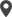Enable Dark Mode!By: Swapna

# Date Utils in Odoo 15

## Technical Odoo 15

Date_utils provides a solid extension to the quality DateTime module. This is often used for getting ranges and references to different time periods, computing relative deltas (next month, next year, next Monday, last week of the month), also, computing relative deltas between two dates, and so forth.
Here, we are going to discuss basic utils in odoo15. First of all, we need to import the package date_utils into the module.
from odoo.tools import date_utils
After the installation/importing of the package, we can use the defined functions of date_utils.
Let’s have a look at the below example.
today = fields.Datetime.now()
This field returns the current date and time. We can take this value as a parameter to pass within the default functions of the basic date utils.
So, Next, have a look at the different default functions of date utils. We already imported the date_utils package from “odoo.tools” . we are passing the value “today” as an argument.
1. get_month()
Eg :  get_month = date_utils.get_month(today)
It returns the range from the first day of the month to the last.
2. get_quarter()
Eg :  get_quarter = date_utils.get_quarter(today)
It returns the range from the first day of the fiscal quarter to the last.
3. get_quarter_number()
Eg: quarter_number = date_utils.get_quarter_number(today)
It returns the number of the current quarter.
4. get_fiscal_year()
Eg: fiscal_year = date_utils.get_fiscal_year(today)
It returns the range from the first day of the fiscal year to the last.
5. start_of()
Eg:
`starting_hour = date_utils.start_of(today, "hour")starting_day = date_utils.start_of(today, "day")starting_week = date_utils.start_of(today, "week")starting_month = date_utils.start_of(today, "month")starting_quarter = date_utils.start_of(today, "quarter")starting_year =date_utils.start_of(today, "year")`
we are using “start_of” functions of hour, day, week, month, quarter, and year. Here also we are passing “today” as the reference parameter. So we can get the starting hour, day, week, month, quarter, and year of today(current day and time).
6. end_of()
Eg:
`ending_hour = date_utils.end_of(today, "hour")ending_day = date_utils.end_of(today, "day")ending_week = date_utils.end_of(today, "week")ending_month = date_utils.end_of(today, "month")ending_quarter = date_utils.end_of(today, "quarter")ending_year =date_utils.end_of(today, "year")`
we are using “end_of” functions of hour, day, week, month, quarter, and year. Here also we are passing “today” as the reference parameter. So we can get the ending hour, day, week, month, quarter, and year of today(current day and time).
We can do calculations using date_utils. Mainly addition and subtraction of days, weeks, months, and years.
Let’s have a look,
As we all know ‘today’ implies the current date and time. If we want to add five days with today, we can use the above expression. We can add as many days as can. We just need to mention the correct number of days. Here we are passing two arguments. One is, the current date and the second is the number of days would wish to add.
If we want to add two weeks with today, use the above example. Here we are passing two arguments. One is the current date, and the second is the number of weeks you would wish to add.
The above expression indicates the addition of one month with today. The parameters are the current date and the number of months you would wish to add.
The above expression indicates the addition of one year with today. The parameters are the current date and the number of years would wish to add.
This expression is used to add days, months, and years together. Need to mention the required number of days, months, and years to be added.
8. subtract()
a) date_utils.subtract(today, days=5)
Passing the parameter number of days to be subtracted from the current date and time.
b) date_utils.subtract(today, weeks=2)
Passing the number of weeks to be subtracted from the current date and time.
c) date_utils.subtract(today, months=1)
Passing the number of months to be subtracted from the current date and time.
d) date_utils.subtract(today, years=1)
Passing the number of years to be subtracted from the current date and time.
e) date_utils.subtract(today, days=2, months=6, years=1)
This will help you to subtract days, months, and years from the current day and time together.
These all are the defined functions of a date utils in odoo.

If you need any assistance in odoo, we are online, please chat with us.

### Recent Posts

#### How to Configure Search View, Filter & Group by in Odoo 17Calicut

Cybrosys Technologies Pvt. Ltd.
Neospace, Kinfra Techno Park
Kakkancherry, Calicut
Kerala, India - 673635London

Cybrosys Limited
Alpha House,
100 Borough High Street, London,
SE1 1LB, United KingdomKochi

Cybrosys Technologies Pvt. Ltd.
1st Floor, Thapasya Building,
Kochi, India - 682030.Bangalore

Cybrosys Techno Solutions
The Estate, 8th Floor,